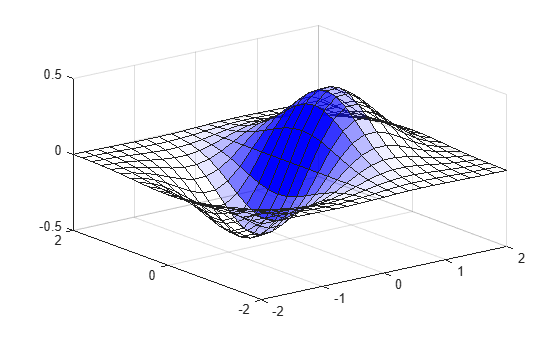# alim

Set or query axes alpha limits

## Syntax

```alpha_limits = alim alim([amin amax]) alim_mode = alim('mode') alim('alim_mode') alim(ax,...) ```

## Description

`alpha_limits = alim` returns the alpha limits (`ALim` property) of the current axes.

`alim([amin amax])` sets the alpha limits to the specified values. `amin` is the value of the data mapped to the first alpha value in the alphamap, and `amax` is the value of the data mapped to the last alpha value in the alphamap. Data values in between are linearly interpolated across the alphamap, while data values outside are clamped to either the first or last alphamap value, whichever is closest.

`alim_mode = alim('mode')` returns the alpha limits mode (`ALimMode` property) of the current axes.

`alim('alim_mode')` sets the alpha limits mode on the current axes. `alim_mode` can be

• `auto` — MATLAB® automatically sets the alpha limits based on the alpha data of the objects in the axes.

• `manual` — MATLAB does not change the alpha limits.

`alim(ax,...)` operates on the specified `Axes` object or `GeographicAxes` object.

## Examples

collapse all

Plot a surface using the gradient of `z` as the alphamap. Adjust the alpha limits to see only where the gradient is between 0 and 0.15.

```[x,y] = meshgrid(-2:.2:2); z = x.*exp(-x.^2-y.^2); surf(x,y,z+.001,'FaceAlpha','flat',... 'AlphaDataMapping','scaled',... 'AlphaData',gradient(z),... 'FaceColor','blue'); alim([0 .15])```## Version History

Introduced before R2006a